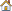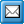﻿ Calculation
QPR Knowledge Base 2017.1

CalculationMetrics User's Guide > Using QPR Metrics > Formulas:

CalculationCalculationParameters for Formulas

A formula can have zero to unlimited number of parameters. Parameters can be constants, NULL values, expressions, wildcard patters, functions, arithmetic or logical operations, or any combination of these. See the Type Conversions section below for information about how different values behave in conversion situations, as especially formulas where logical and arithmetic operations are combined can produce unexpected results if the characteristics of the value conversions are not taken into account.

Note: Formula parameters are not case sensitive. Therefore, the formula parameters can be entered in both uppercase and lowercase letters.

Note: Text series can be used in textual and numeric formulas instead of textual constants with the following limitations:

Symbolic references that return several series are supported only if either all returned series are numeric, or if only one textual series is returned. That is, symbolic references returning string lists are not supported, nor are references that return mixed lists. For example:

SUM_(?.ACT()) works OK if ?.ACT() is resolved to numeric values, such as "1, 2, 3".

SUM_(?.ACT()) will return an error if ?.ACT() is resolved to numeric values "1, 2" and a textual value "3" (all returned series are not numeric).

SUM_(?.ACT()) will return an error if ?.ACT() returns two textual values: "1" and "2" (string lists are not supported)

Note: As a general rule, text and numeric series can be mixed freely in formulas. If the usage expects a numeric value and the series is textual, the calculation engine tries to convert the text value into a number. If it doesn't succeed, an error is returned. In addition, when string processing formulas are applied to a number, the calculation engine converts the number to text before calculation.

Type Conversions

All QPR Metrics formula results, except those coming from string processing formulas, are always converted to numbers. The following table shows all possible conversions and how they are handled in the calculation engine.

From

To

Conversion method

Number

Number list

Produces a number list filled with the number.

Number

String

Produces number presented as a string.

Number

Boolean

0 is converted to "false", and all other values are converted to "true".

Number list

Number

The result is a number which is the first number in the number list.

Number list

String

Produces a string containing all numbers in a number list separated by spaces.

String

Number

Produces a number, which is the first number in the string.

String

Number list

Produces a number list from the numbers in the string.

Boolean

Number

"False" is converted to 0 and "true" is converted to anything else than 0. The actual result value depends on the context in which the operation is performed.

Automatic Recalculation

A measure (an element) can have values which are derived from some other measure values. To prevent situations where dependent measure values are not up to date, any change in any measure value triggers a recalculation which will guarantee the coherence of a model.

The following events will trigger the automatic recalculation:

Event

Actions

Element's value changed

Recalculation of the related period.

Element's value deleted

Recalculation of the related period.

Recalculation of the related period.

Element's value settings changed

Verification for recalculating all periods is requested. If user confirms the recalculation, all related elements will be updated.

New formula created

Verification for recalculating all periods is requested. If user confirms the recalculation, all related series will be updated.

Formula changed

Verification for recalculating all periods is requested. If user confirms the recalculation, all related series will be updated.

New period created

All elements the formulas of which have a reference to the created period, will be updated.

Element identifier changed

If any formula is using the related identifier, verification for recalculating all periods will be requested. If user confirms the recalculation, all series utilizing the related element, through wildcard pattern(s), will be updated.

Range value changed

Verification for recalculating all periods is requested. If user confirms the recalculation, the related model will be updated.

None.

Element moved

Verification for recalculating all periods is requested. If user confirms the recalculation, previous and current parent elements, utilizing wildcard patterns, will be updated.

Element deleted

Verification for recalculating all periods is requested. If user confirms the recalculation, all related elements will be updated.

Scorecard identifier changed

Verification for recalculating all periods is requested. If user confirms the recalculation, all elements utilizing the identifier through wildcard patterns will be updated.

Value Accumulation

When elements form a hierarchical structure of parent and child elements, parent elements often need to accumulate values from their child elements. If a parent element has a longer period level than its child elements, conversion is needed for accumulating the values.